# Why “df.to_csv” could be a Mistake ?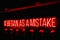Photo by Varvara Grabova on Unsplash

As a Data Scientist and in the field of data analysis more globally, load and save data (DataFrame) is almost systematic.

Usually, I use `df.to_csv('path/df.csv')` and i think that almost all Pandas users do the same or did that at least once.

All Python users know that saving data in CSV format is very practical and very easy but it has some drawbacks, which we will detail below, that can turn this usage as a bad habit.

For the rest of this article, I will generate a Pandas DataFrame, as follow, and I will use it as an example.

`from uuid import uuid4import numpy as npimport pandas as pddef generate_strings(n_rows, n_cols, taux_nan):    """        This function is used to generate a string variables    """    df_ = pd.DataFrame()    for col in range(n_cols):        name = f'str_{col}'        cats = [str(uuid4()) for _ in range(n_rows)]        values = np.array(cats, dtype=object)        nan_cnt = np.random.randint(1, int(taux_nan*n_rows))        index = np.random.choice(n_rows, nan_cnt, replace=False)        values[index] = np.nan        df_[name] = values    return df_def generate_numeric(n_rows, n_cols, taux_nan):    """        This function is used to generate a numeric variables    """    df_ = pd.DataFrame()    for col in range(n_cols):        name = f'num_{col}'        nums = np.random.normal(0, 1, n_rows)        nan_cnt = np.random.randint(1, int(taux_nan*n_rows))        index = np.random.choice(n_rows, nan_cnt, replace=False)        nums[index] = np.nan        df_[name] = nums    return df_def generate_dates(n_rows, n_cols, taux_nan):    """        This function is used to generate a dates variables    """    df_ = pd.DataFrame()    for col in range(n_cols):        name = f'date_{col}'        dates_initial = pd.date_range(start='2010-01-01', end='2021-                                                              03-01')        dates = np.random.choice(dates_initial, n_rows,                                                           replace=True)        nan_cnt = np.random.randint(1, int(taux_nan*n_rows))        index = np.random.choice(n_rows, nan_cnt, replace=False)        dates[index] = None        df_[name] = dates    return df_def generate_categories(n_rows, n_cols, taux_nan):    """        This function is used to generate a categorical variables    """    df_ = pd.DataFrame()    for col in range(n_cols):        name = f'cat_{col}'        cats = [str(uuid4()) for _ in range(n_rows)]        values = pd.Categorical(cats)        nan_cnt = np.random.randint(1, int(taux_nan*n_rows))        index = np.random.choice(n_rows, nan_cnt, replace=False)        values[index] = np.nan        df_[name] = values    return df_def generate_df():    """        This function is used to generate all data        -------        Returns : Pandas dataframe    """    n_rows, n_cols, taux_nan = 100000, 6, 0.05    df_string = generate_strings(n_rows, n_cols, taux_nan)    df_nums = generate_numeric(n_rows, n_cols, taux_nan)    df_dates = generate_dates(n_rows, 2, taux_nan)    df_cats = generate_categories(n_rows, n_cols, taux_nan)    df_ = pd.concat([df_string, df_nums, df_dates, df_cats], axis=1)    return df_df = generate_df()`

1. Save data as CSV file don’t save format

You have already imported CSV files and noticed that columns formats have disappeared.
DateTime columns are casted into String columns, categorical columns are casted into String…

See an example in the following picture.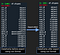CSV export effect on variables types

And there, you have to cast them to the right type and waste a crazy time on extra worthless task that can be avoided by using other backup format.

2. Size of the CSV File can be reduce by 16 times

The second reason why you need to change this habit of exporting DataFrames as CSV is the size of the exported .CSV files.

Here we will compare, in terms of file size, two export formats CSV and Parquet using 4 DataFrames.

We will compare the following DataFrame with the same column number but different row number: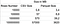The greater row number, the greater the difference between the two files
`df.to_csv('./results/df.csv', index=False) #We save our df using csvdf.to_parquet('./results/df.parquet', compression='gzip') #We save same df using Parquet`

So next we plot the above results to see the difference between the two formats that we compare.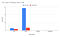Plot of files size for the two first DataFrame (10 000 rows and 100 000 rows)

Here we plot the size of only the two first DataFrame (DataFrame with
10 000 rows and 100 000 rows).

The difference between the two format, CSV and Parquet, is obvious.

Files saved in CSV format is much bigger than Parquet format.

Now, we plot the size of all DataFrame (we add DataFrame with 1 000 000 rows and
100 000 000 rows) and here the difference is even more striking.

To conclude, when files are small (of the order of a few thousand rows) there is not a big difference between the two formats but when the number of rows increases the difference becomes more and more significant.

Advice: Choose Parquet format systematically when the row number is >10k.

3. CSV Format Takes Too Much Time When Exporting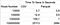Time to save DataFrame using CSV et Parquet format

There, we have the same conclusion that above, when the file size is small, around 10 000 rows, the time to save a DataFrame using CSV or Parquet format is the same but the time to save a DataFrame in CSV format is much more important than the time to save a file using Parquet format.

Advice: Choose Parquet format systematically when the row number is >10k.

4. Index Management

When you use other format like Parquet, you don’t need to add `index=False` to avoid exporting DataFrame Index.

## Conclusion:

It’s obvious that CSV format isn’t the best format to store data but that doesn’t mean that the Parquet format is the best one to be used.

The reason that CSV is not the best solution are:

• CSV don’t save the Data type (DateTime column convert to String for example)
• CSV size is huge
• Time to save CSV is huge
• Index management for CSV format

The are a lot of other format that can be used as an alternative of CSV format: HDF format, feather format, pickle format …

## Analytics Vidhya

Analytics Vidhya is a community of Analytics and Data…

## Sign up for Analytics Vidhya News Bytes

### By Analytics Vidhya

Latest news from Analytics Vidhya on our Hackathons and some of our best articles! Take a look.

By signing up, you will create a Medium account if you don’t already have one. Review our Privacy Policy for more information about our privacy practices.

Check your inbox
Medium sent you an email at to complete your subscription.

Written by

## Elfao

Data scientist with 4 years experience.

## Analytics Vidhya

Analytics Vidhya is a community of Analytics and Data Science professionals. We are building the next-gen data science ecosystem https://www.analyticsvidhya.com

Written by

## Elfao

Data scientist with 4 years experience.## Analytics Vidhya

Analytics Vidhya is a community of Analytics and Data Science professionals. We are building the next-gen data science ecosystem https://www.analyticsvidhya.com

Medium is an open platform where 170 million readers come to find insightful and dynamic thinking. Here, expert and undiscovered voices alike dive into the heart of any topic and bring new ideas to the surface. Learn more

Follow the writers, publications, and topics that matter to you, and you’ll see them on your homepage and in your inbox. Explore

If you have a story to tell, knowledge to share, or a perspective to offer — welcome home. It’s easy and free to post your thinking on any topic. Write on Medium